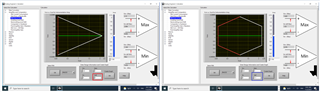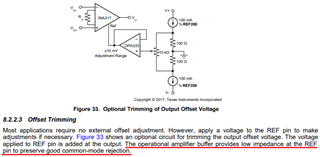If you have a related question, please click the "Ask a related question" button in the top right corner. The newly created question will be automatically linked to this question.

# INA317: performance with power supply below 3.3V

Part Number: INA317
Other Parts Discussed in Thread: INA333

Hi team,

I found the statement below on the datasheet "Power Supply Recommendations". Which specification will be reduced by using power supply below 3.3V e.g. 2.2V?

I saw the input common-mode range and output voltage are depends on the supply voltage, but I want to know anything else.

"The minimum power supply voltage for INA317 is 1.8 V and the maximum power supply voltage is 5.5 V. For optimum performance, 3.3 V to 5 V is recommended."

Regards,
Kazuto

• Hi Kazuto-san,

Which specification will be reduced by using power supply below 3.3V e.g. 2.2V?

INA317 and INA333 have the identical die package; the only differences are in its Vos specification.

If the application is using 2.2Vdc in a single supply rail, the Vcm and Vout dependency are shown on the left graph, where Vref = 0V.

If the Vref is configured at the mid rail of 2.2Vdc, then the Vcm and Vout dependency are shown on the right graph, see the image below. Please note that Vcm and Vout ranges between two configurations, which the highlighted enclosed area in the graph are operating in INA317's linear region.https://www.ti.com/tool/ANALOG-ENGINEER-CALC

The Vref pin in INA317 is required to be a low impedance node and is required to be able to source or sink in current. Below is a suggested reference design. You may use resistor divider to generate the Vref voltage, say 1.1Vdc. You may use suggested current source or similar circuit to generate the Vref voltage, if high precision is required.Best,

Raymond

• Hi Raymond,

Thank you for your answer. I understood that the Vout will be saturated if the input common mode voltage is high and Vs is low, is that correct?

Additionally, I see the characteristics listed below is affected by Vs. If there is anything else please let me know.

• Figure 5. Offset Voltage vs Common-Mode Voltage
• Figure 18. Common-Mode Rejection Ratio vs Temperature
• Figure21, Figure 23. Typical Common-Mode Range vs Output Voltage
• Figure 26. Input Bias vs Temperature
• Figure 27. Input Bias Current vs Common-Mode Voltage
• Figure 28. Input Offset Current vs Temperature
• Figure 29. Output Voltage Swing vs Output Current
• Figure 30. Quiescent Current vs Temperature
• Figure 31. Quiescent Current vs Common-Mode Voltage

Regards,
Kazuto

• Hi Kazuto,

if the input common mode voltage is high and Vs is low, is that correct?

The input common mode voltage and Vout signal have to inside of the boundaries in order to have the linear responses per the instrumentation amplifier in the given conditions, see the chart below. The Analog Engineer Calculator is duplicate the plots shown in Figure 20, 21, 22 & 23, but the following plots are  shown the specific Vcm and Vout relationships with single supply voltage of 2.2Vdc with and without Vref voltage.If you are able to provide us the input parameters, I can put together a simulation for you.

Best,

Raymond